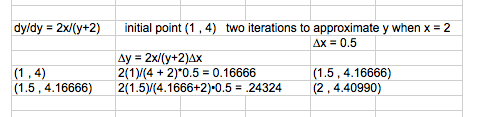## Euler’s Method

We are now going to add one more method to solving differential equations: Euler’s Method.

Euler’s Method is a numerical method of approximation of the solution to a differential equation by computing points on the solution curve. In some cases we can’t find an equation for the curve, but we can always find a graphical solution (slope fields) or a numerical solution (Euler’s Method). It essentially writes a series of tangent lines close together to create the solution.

We need a differential equation (dy/dy = ?) , a starting point (x , y) and either the number of iterations and an ending x value (we then compute the ∆x ) or given ∆x and the number of iterations (we then compute the ending x-value).

With each iteration, we move right (or left) by our ∆x and we move up some ∆y =  dy/dy•∆x, where dy/dy is the slope of the line at the point (x , y) and we know ∆x.  We establish our new point as (x + ∆x , y + ∆y) and complete the necessary iterations to give us a final value. This series of iterations gives us an approximation for the solution to a differential equation given an initial condition. We many not be able to find an algebraic solution to the differential equation, but we can always give a numerical solution (Euler’s Method) or graphical solution (Slope Field).

#### dy/dx = y/(x+1) initial point (2 , -1) two iterations to approximate y when x = 2.5#### dy/dx = 2x/(y+2) y(1) = 4, compute 2 iterations with ∆x = 0.5 to approximate y(2)